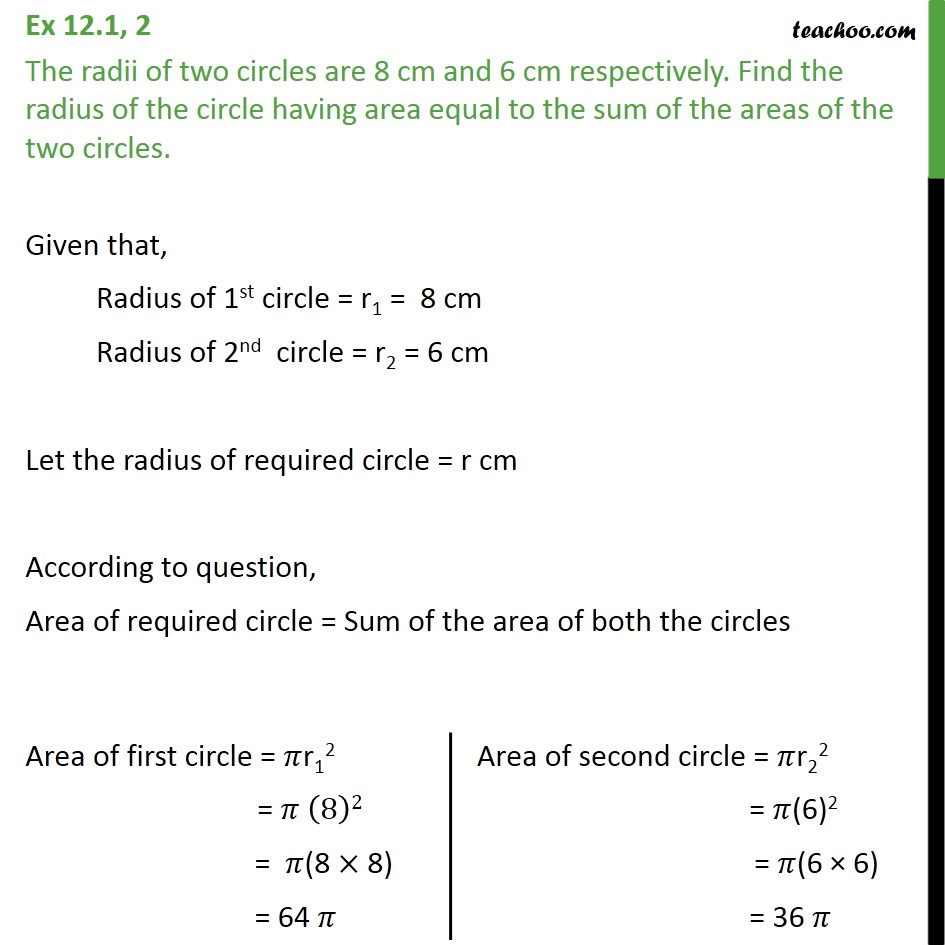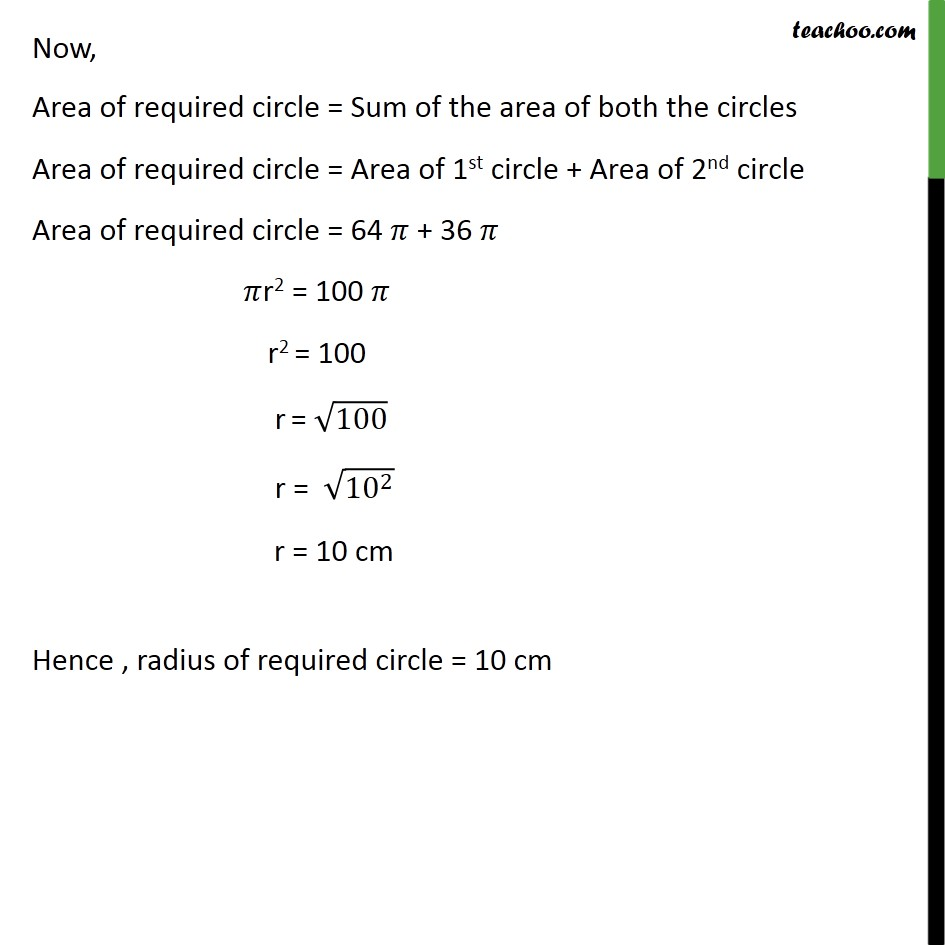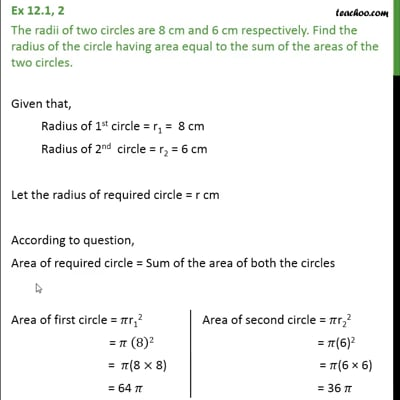Ex 12.1

Chapter 12 Class 10 Areas related to Circles
Serial order wiseThis video is only available for Teachoo black users

Solve all your doubts with Teachoo Black (new monthly pack available now!)

### Transcript

Ex 12.1, 2 The radii of two circles are 8 cm and 6 cm respectively. Find the radius of the circle having area equal to the sum of the areas of the two circles. Given that, Radius of 1st circle = r1 = 8 cm Radius of 2nd circle = r2 = 6 cm Let the radius of required circle = r cm According to question, Area of required circle = Sum of the area of both the circles Now, Area of required circle = Sum of the area of both the circles Area of required circle = Area of 1st circle + Area of 2nd circle Area of required circle = 64 𝜋 + 36 𝜋 𝜋r2 = 100 𝜋 r2 = 100 r = √100 r = √(〖10〗^2 ) r = 10 cm Hence , radius of required circle = 10 cm